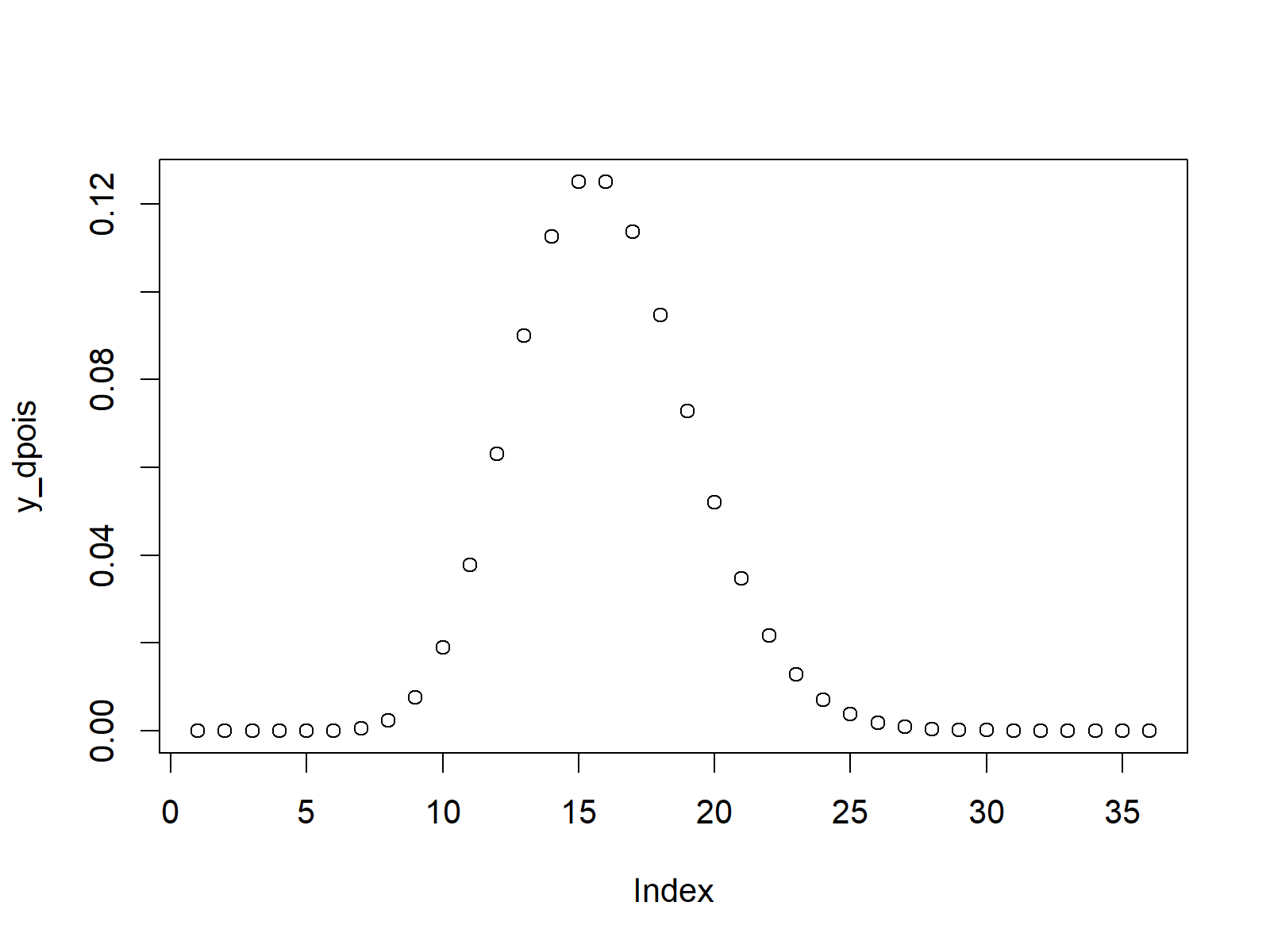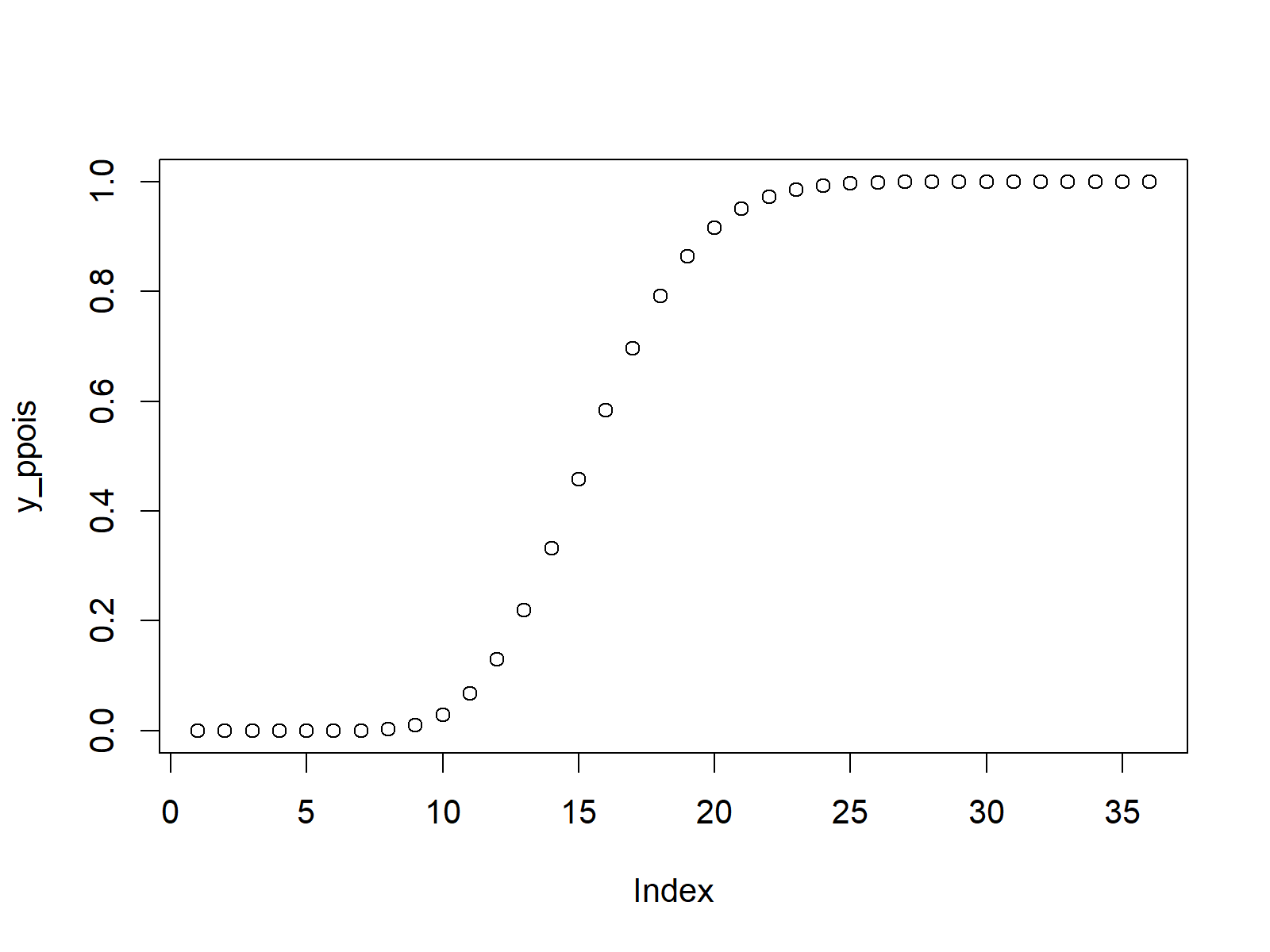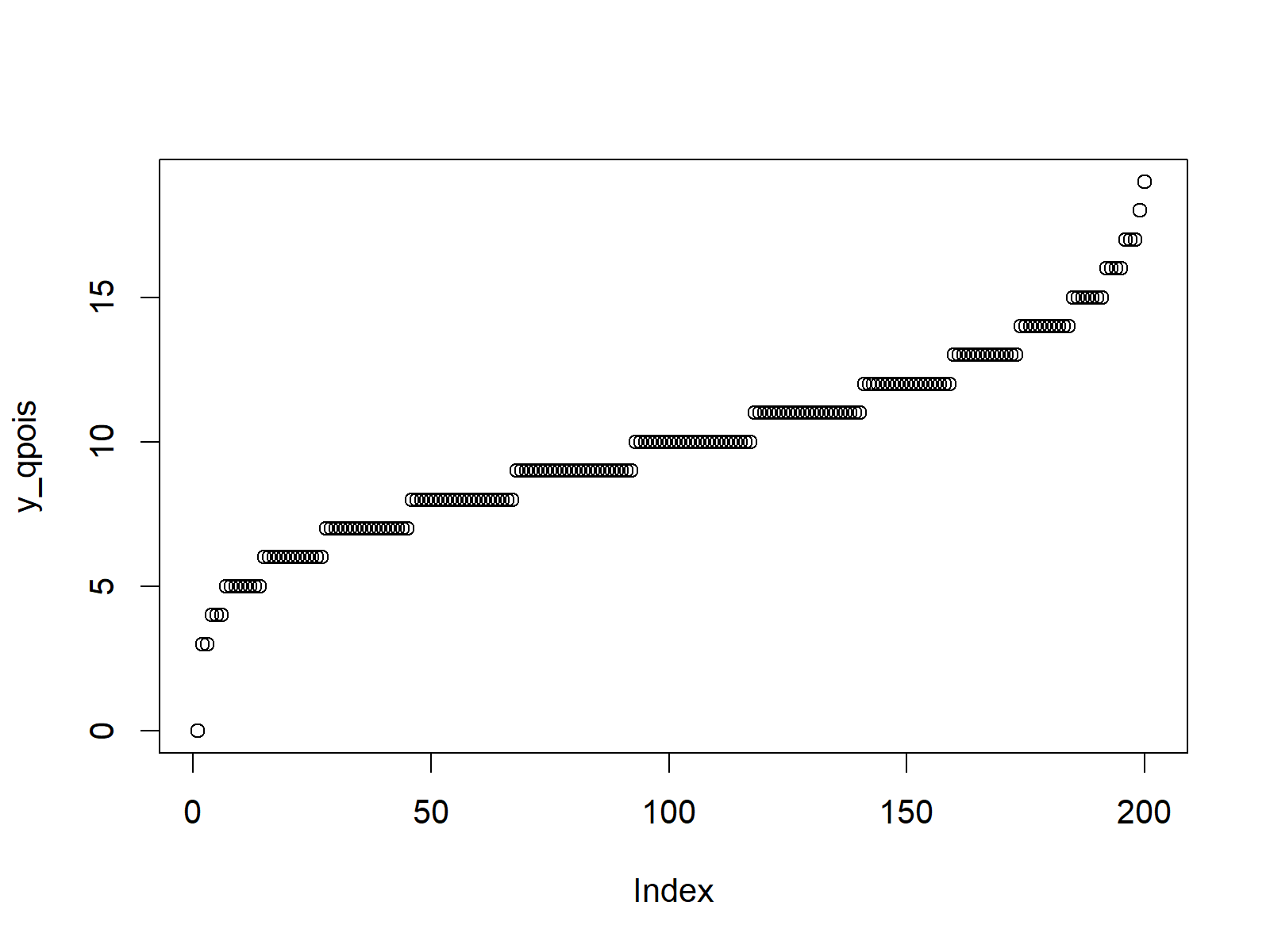# Poisson Distribution in R (4 Examples) | dpois, ppois, qpois & rpois Functions

In this R tutorial you’ll learn how to use the poisson functions.

The content is structured as follows:

Let’s get started…

## Example 1: Poisson Density in R (dpois Function)

This example shows the poisson density illustrated in an R plot.

In order to create a poisson density in R, we first need to create a sequence of integer values:

`x_dpois <- seq(- 5, 30, by = 1)                    # Specify x-values for dpois function`

Now we can return the corresponding values of the poisson density for each of these values. In the example, we use a lambda of 10:

`y_dpois <- dpois(x_dpois, lambda = 10)             # Apply dpois function`

If we want to create a graph showing these probability density values, we can apply the plot function:

`plot(y_dpois)                                      # Plot dpois values`Figure 1: Poisson Density in R.

## Example 2: Poisson Distribution Function (ppois Function)

In the second example, we will use the ppois R command to plot the cumulative distribution function (CDF) of the poisson distribution.

Again, we first need to specify a vector of values, for which we want to return the corresponding value of the poisson distribution:

`x_ppois <- seq(- 5, 30, by = 1)                    # Specify x-values for ppois function`

We then can apply the ppois function…

`y_ppois <- ppois(x_ppois, lambda = 10)             # Apply ppois function`

…and create an R plot as follows:

`plot(y_ppois)                                      # Plot ppois values`Figure 2: Poisson Distribution in R.

## Example 3: Poisson Quantile Function (qpois Function)

Similar to the previous examples, we can also create a plot of the poisson quantile function. Let’s create a sequence of values to which we can apply the qpois function:

`x_qpois <- seq(0, 1, by = 0.005)                   # Specify x-values for qpois function`

Now, we can apply the qpois function with a lambda of 10 as follows:

`y_qpois <- qpois(x_qpois, lambda = 10)             # Apply qpois function`

With the plot function, we can illustrate our output:

`plot(y_qpois)                                      # Plot qpois values`Figure 3: Poisson Quantile Function in R Plot.

## Example 4: Random Number Generation (rpois Function)

In case we want to draw random numbers according to the poisson distribution, we can use the following R code.

First, we need to specify a seed to ensure reproducibility and a sample size of random numbers that we want to draw:

```set.seed(13579)                                    # Set seed for reproducibility
<pre lang="csharp">N <- 10000                                         # Specify sample size```

Then, we can apply the rpois functions as shown below:

```y_rpois <- rpois(N, lambda = 10)                   # Draw N poisson distributed values
y_rpois                                            # Print values to RStudio console
# 6 14  8 16  6 12 10  6  7 11  7 12 10 16  7  7  7 19 13```

As you can see based on the RStudio output, the rpois function returned a set of random integer numbers. We can show these random numbers in a histogram with the hist function:

```hist(y_rpois,
breaks = 100,
main = "Poisson Distribution in R")           # Plot histogram of rpois values```Figure 4: Randomly Generated Histogram of Poisson Distribution.

## Video & Further Resources

Have a look at the following video of my YouTube channel. In the video, I’m explaining the R syntax of this article:

You may also read the other posts on distributions and the simulation of random numbers in R:

Besides that, you could have a look at the related tutorials of https://statisticsglobe.com/. Some tutorials about different types of distributions can be found here:

In this article you learned how to draw and simulate a poisson distribution in the R programming language. Let me know in the comments, in case you have any further questions.

Subscribe to the Statistics Globe Newsletter